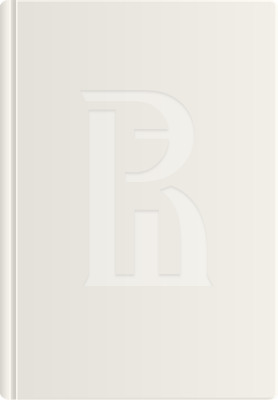• A
• A
• A
• ABC
• ABC
• ABC
• –ź
• –ź
• –ź
• –ź
• –ź
Regular version of the site

## Lectures on universal TeichmuŐąller space

Sergeev A.

This book is based on a lecture course given by the author at the Educational Center of the Steklov Mathematical Institute in 2011. It is designed for a one-semester course for undergraduate students familiar with basic differential geometry and complex and functional analysis.

The universal Teichmüller space T is the quotient of the space of quasisymmetric homeomorphisms of the unit circle modulo Möbius transformations. The first part of the book is devoted to the study of geometric and analytic properties of T. It is an infinite-dimensional Kähler manifold which contains all classical Teichmüller spaces of compact Riemann surfaces as complex submanifolds, which explains the name "universal Teichmüller space". Apart from classical Teichmüller spaces, T contains the space S of diffeomorphisms of the circle modulo Möbius transformations. The latter space plays an important role in the quantization of the theory of smooth strings.

The quantization of T is presented in the second part of the book. In contrast with the case of diffeomorphism space S, which can be quantized in frames of the conventional Dirac scheme, the quantization of T requires an absolutely different approach based on the noncommutative geometry methods.

The book concludes with a list of 24 problems and exercises which can used to prepare for examinations.